Other Parts Discussed in Post: AMC1304M25

The AMC1304 reinforced isolated modulator family is designed specifically for systems, such as motor controllers and solar power inverters, where the presence of high voltage demands the use of an isolation barrier in order to protect both the end user and sensitive components. Because of this isolation barrier requirement, you’ll need two power supplies: one for the analog input side (also referred to as the high side) and another for the digital interface side (also referred to as the controller side).

On the controller side, many isolated acquisition systems have a 3.3-V DC supply; on the other side of the isolation barrier, however, there may not be a suitable power supply for the AMC1304. Thankfully, there are some ways to get around this.

Zener diodes provide the simplest solution in a system where a DC-floating power supply is available. Figure 1 shows an example of this approach.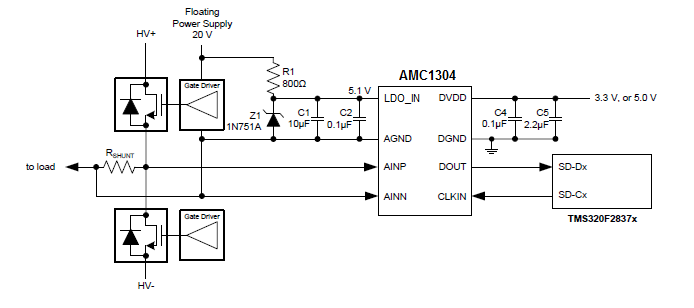Figure 1: Zener-diode-based high-side power supply

You can achieve a more energy-efficient solution in multichannel systems by using the same approach described in this article. That approach consists of a step-down switching regulator (Figure 2) followed by multiple copies of push-pull drivers (Figure 3).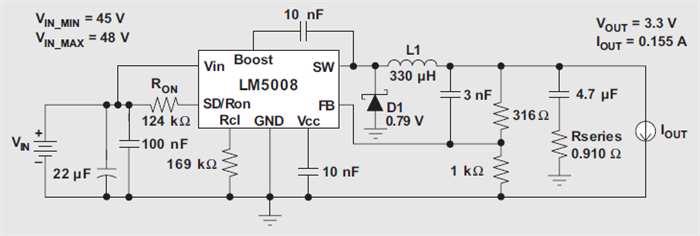Figure 2: Step-down switching regulator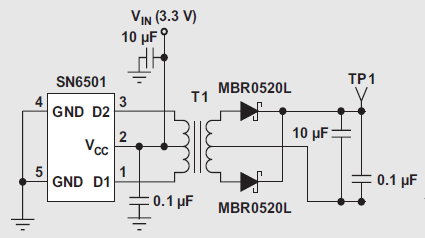Figure 3: Isolated supply from 3.3V DC

If the system uses only one sensing channel, then you can use the power supply shown in Figure 4.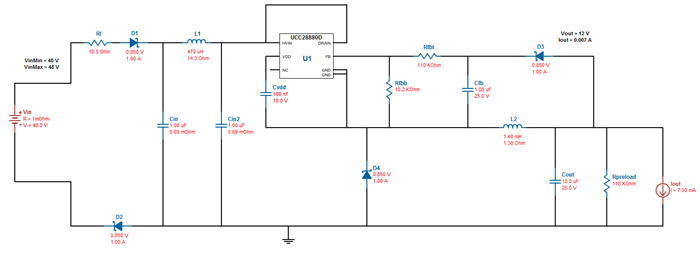Figure 4: Efficient power supply option for a single-channel system

You can obtain and modify the power-supply solutions shown in Figures 2, 3 and 4 through TI’s WEBENCH® design and simulation tools.

To monitor variables in AC power lines, capacitive-drop (cap-drop) power supplies are typically less expensive than their switching-mode counterparts. Figure 5 shows a cap-drop supply for the AMC1304 family.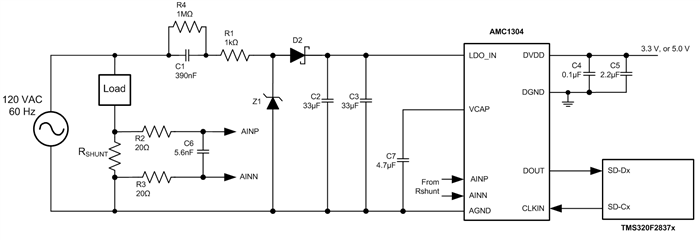Figure 5: Cap-drop supply for AMC1304 family

Designing this type of supply is relatively straightforward. Choose the value of C1 from the load current (in this case the maximum AVDD supply current to the LDO_IN pin of the AMC1304 family), the peak line voltage (assuming that the line voltage is sinusoidal at 120VRMS) and the line voltage frequency (60Hz), as shown in Equation 1:

Equation 2 shows how to calculate the value of the capacitor connected to the low-dropout (LDO) input of the AMC1304 family for a given maximum LDO input ripple (ΔVMax). In Figure 5, this capacitance is obtained through the parallel combination of C2 and C3.

In Figure 5, Z1 is a 12-V Zener diode that establishes the maximum LDO input voltage (for example, the BZT52C12S Zener diode can be used for Z1). In Figure 5, D2 can be a Schottky diode with low forward voltage and more than 20V of reverse breakdown voltage (for example, the DB2J31000L and CDBU0230 Schottky diodes can be used for D2). In Figure 5, R4 is included for safety purposes in order to discharge C1 when the system is de-energized, while R1 prevents a high inrush current when the system is hot-plugged to the power line.

If you’re interested in cap-drop supplies, this application report shows an interesting design with step-by-step instructions.

Several other options exist for supplying the high side of isolated delta-sigma modulators. Although some exploit high-performance switching-mode integrated circuits (ICs), you can also use a small number of simple components to create cost-effective solutions.

How will you supply your isolated modulator?